# Mellin transform

(diff) ← Older revision | Latest revision (diff) | Newer revision → (diff)

The integral transform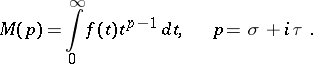The substitutionreduces it to the Laplace transform. The Mellin transform is used for solving a specific class of planar problems for harmonic functions in a sectorial domain, of problems in elasticity theory, etc.

The inversion theorem. Suppose that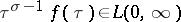and that the functionhas bounded variation in a neighbourhood of the point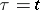. ThenThe representation theorem. Suppose that the functionis summable with respect toon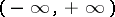and has bounded variation in a neighbourhood of the point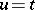. Then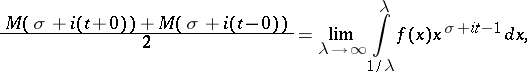where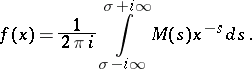How to Cite This Entry:
Mellin transform. Encyclopedia of Mathematics. URL: http://encyclopediaofmath.org/index.php?title=Mellin_transform&oldid=15680
This article was adapted from an original article by P.I. Lizorkin (originator), which appeared in Encyclopedia of Mathematics - ISBN 1402006098. See original article